# User:IssaRice/Tao's notation for limits

Tao's notation for a limit is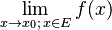$\lim_{x\to x_0;\, x\in E} f(x)$.

Can we write this in a more standard way? basically, if we give one additional definition, we have the very appealing formula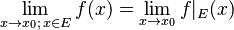$\lim_{x\to x_0;\, x\in E} f(x) = \lim_{x\to x_0} f|_E(x)$.

The additional definition is this: if$f : X \to \mathbf R$, then we define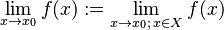$\lim_{x\to x_0} f(x) := \lim_{x\to x_0;\, x \in X} f(x)$. In other words, by default we assume that the limit is taken over the entire domain of the function.

Now, given$f : X \to \mathbf R$ and some$E \subseteq X$, we have$f|_E : E \to \mathbf R$. Thus,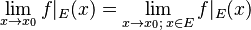$\lim_{x\to x_0} f|_E(x) = \lim_{x\to x_0;\, x \in E} f|_E(x)$.

By exercise 9.4.6,$\lim_{x \to x_0;\, x\in E} f(x) = \lim_{x\to x_0;\, x\in E} f|_E(x)$

Combining these two equalities, we have$\lim_{x \to x_0;\, x\in E} f(x) = \lim_{x\to x_0} f|_E(x)$ as promised.

Rather than thinking of this as a result per se, I think it's better to think of this as alternative notations. Why might one prefer one notation over the other? I think the strength of Tao's notation is that it works for anonymous functions/expressions. To be able to use the function restriction notation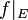$f|_E$, we must have named our function beforehand. To give an example, we can write something like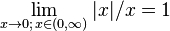$\lim_{x\to 0;\, x\in (0,\infty)} |x|/x = 1$, but this is difficult to write in the other notation; we would have to say something like, "Let$f:\mathbf R\setminus \{0\} \to \mathbf R$ be defined by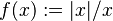$f(x) := |x|/x$. Then we have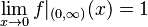$\lim_{x\to 0} f|_{(0,\infty)}(x) = 1$."

An amusing fact/potential advantage of the function restriction notation is that one can write it in a point-free notation. Namely, we can write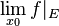$\lim_{x_0} f|_E$. Actually, even with Tao's notation we could have written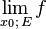$\lim_{x_0;\, E} f$.

I think usually one would write the above like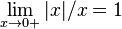$\lim_{x\to 0+} |x|/x = 1$. So then one gets to keep anonymous functions, but at the expense of adding more complexity to the notation (one now needs to assign meaning to "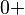$0+$").

One should compare this to the function notation ($f'$) vs Leibniz notation (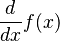$\frac{d}{dx} f(x)$) for derivatives.

Notation Symbols Strengths Weaknesses
Tao's notation$\lim_{x\to x_0;\, x\in E} f(x)$ Works with anonymous functions. Somewhat verbose: the "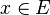$x\in E$" part must be written out each time.
Function restriction notation$\lim_{x\to x_0} f|_E(x)$ Consistent with the standard notation. Doesn't work with anonymous functions.
Standard notation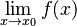$\lim_{x\to x_0} f(x)$ Works with anonymous functions. Set used in the limit does not appear in the notation, so one must assume some default set (such as the entire domain of the function) or introduce new notation for e.g. one-sided limits as e.g.$\lim_{x\to x_0+} f(x)$ and$\lim_{x\to x_0-} f(x)$.